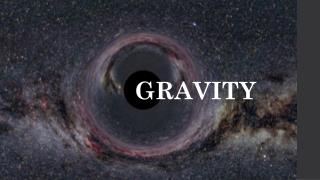DownloadDownload PresentationGRAVITY

GRAVITY

Download PresentationGRAVITY

- - - - - - - - - - - - - - - - - - - - - - - - - - - E N D - - - - - - - - - - - - - - - - - - - - - - - - - - -
Presentation Transcript

1. GRAVITY

2. A Brief Timeline of the Discovery of Gravity

3. Aristotle 4TH Century BC There is no effect or motion without a cause. The element of earth was thought to be heavy by nature and therefore pulled towards the centre of the (geocentric) universe. Lighter elements on the other hand, such as fire were thought to be pulled towards the moon. Thus in Aristotle's system heavy bodies tend toward each other due directly to the weight of the material, this was known as gravitas.

4. 16TH /17TH Century AD Galileo In his famous experiment he dropped balls from the leaning Tower of Pisa, and later with careful measurements of balls rolling down inclines, Galileo showed that gravitation accelerates all objects at the same rate. This was clearly contrary to Aristotle’s thought that heavier objects accelerate faster. Galileo postulated air resistance as the reason that lighter objects may fall slower in an atmosphere. His work served as a foundation for Newton’s later discovery.

5. 1687 Newton Newton published Principia, which hypothesizes the inverse-square law of universal gravitation. In his own words, “I deduced that the forces which keep the planets in their orbs must [be] reciprocally as the squares of their distances from the centres about which they revolve: and thereby compared the force requisite to keep the Moon in her Orb with the force of gravity at the surface of the Earth; and found them answer pretty nearly.”

6. 1687 Newton It was used to predict the existence of Neptune based on motions of Uranus that could not be accounted for by the actions of the other planets. However, the downfall of the Newtonian theory appeared when the perturbations of Mercury’s orbit could not be explained, paving the way for an alternative explanation.

7. Einstein 1907 In general relativity, the effects of gravitation are ascribed to spacetime curvature instead of a force. The theory starts with the equivalence principle, which equates free fall with inertial motion, and describes free-falling inertial objects as being accelerated relative to non-inertial observers on the ground. In Newtonian physics, however, such acceleration is impossible without a force acting on it.

8. Einstein 1907 Einstein proposed that spacetime is curved by matter, and that free-falling objects are moving along locally straight paths in curved spacetime. These straight paths are called geodesics. For instance, we are no longer following geodesics while standing because the mechanical resistance of the Earth exerts an upward force on us, and we are non-inertial on the ground as a result. This explains why moving along the geodesics in spacetime is considered inertial.

9. Gravity as a force Although gravity was the first force discovered, it is also the most individual of the forces in the way that it is ununified with the other 3 forces. And exhibits different properties If these particles exist then we will have to change our fundamental understanding of the universe in which we live. General relativity predicts that gravity exists in the form of a particle, the graviton.

10. Properties of Gravitons • Gravitons like all force carrier particles have particular characteristics: • - Fundamental particle • Zero mass • Zero charge • Spin of 2 • And is its own antiparticle

11. Gravitational waves Gravitational waves have many similar properties to other types of waves. However gravitational waves are ripples in the curvature of space-time itself. And ultimately distort the dimensions which we live in. There are potentially many sources of gravitational waves which we may detect.

12. Wave/Particle hunting There are currently 2 types of detectors looking for gravitational waves: • Michelson inferometer • Polarization of CMB What’s the difference?

13. Latest Results • Thus far LIGO has not found any concrete evidence of gravitational waves. • BICEP2 has made a recent discovery about gravitational wave ripples from the big bang.

14. Gravitational Interaction:Black HolesBy David Hadden, 5/6/2014 Main points: Formation and general interest, · Event Horizons and the Schwarzschild Radius, · Gravitational Redshift, · Gravitational lensing.

15. Formation and general Interest • First postulated by John Michell in 1783. • Schwarzschild first categorized a black hole from solutions to Einstein’s field equations. • Formed from dying stars. General form of Einstein’s field equations.

16. Event Horizons and the Schwarzschild Radius • An area of space beyond which light cannot escape, • p • Radius of a sphere such that if the mass of the object is condensed into it, the escape velocity is the speed of light. • Sun: 3km • Earth: 9mm

17. Gravitational redshift • Caused due to large deformations in spacetime, • Gravitational time dilation, • Redshifted when observed in a region of lower gravitational field.

18. Gravitational Lensing • From general relativity, light bent by mass, • Acts like an actual lens , and bends light rays towards eye, • Formula, simplifies to, • Observations. = =2 David Hadden, slide 5

19. Thank you for listening!